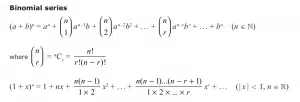# Sequences and Series in A-Level Maths

## Sequences & Series

You have seen some Sequences and Series before. Recall that during GCSE Maths you were taught the nth term for linear and quadratic sequences and you also looked at compound interest.

The difference between a sequence and a series is that the terms in a sequence are listed whereas the terms in series are summed. Of course, you can have an infinite sequence but for an infinite series to exist, the summation must converge. Examples of infinite series include binomial expansions when powers of your binomial are negative or fractional or both. Binomial Expansion is studied in both years of A-Level Maths. Firstly, positive integer powers of the expansion are studied in Year 1 – this is the only topic we study in Sequences and Series in Year 1. Secondly, as mentioned above, negative/fractional powers are studied in Year 2. Note that the formulae for binomial expansions is given in the formula booklet:In addition to binomial expansion with negative/fractional powers, you will study arithmetic and geometric series. In an arithmetic series the terms change by a common factor, whereas in a geometric series, they change by a common factor. You may also see closed form sequences and sigma notation during this second year.